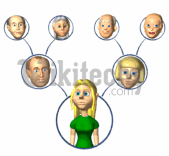# C++ Multiple Inheritance | Hierarchical Inhertiance - Learn C++ - C++ Tutorial - C++ programmingLearn c++ - c++ tutorial - c++-multiple-inheritance - c++ examples - c++ programs

• In this, we will discuss about different models of inheritance in C++ programming: Multiple, Multilevel and Hierarchical inheritance with examples.• Inheritance is one of the core feature of an object-oriented programming language. It allows software developers to derive a new class from the existing class. The derived class inherits the features of the base class (existing class).
• There are various models of inheritance in C++ programming.

## C++ Multilevel Inheritance

• In C++ programming, not only you can derive a class from the base class but you can also derive a class from the derived class. This form of inheritance is known as multilevel inheritance.
•learn c++ tutorials - multiple inheritance in c++ Example

• Here, class B is derived from the base class A and the class C is derived from the derived class B.

## Output

• In this program, class C is derived from class B (which is derived from base class A).
• The obj object of class C is defined in the main() function.
• When the display() function is called, display() in class A is executed. It's because there is no display() function in class C and class B.
• The compiler first looks for the display() function in class C. Since the function doesn't exist there, it looks for the function in class B (as C is derived from B).
• The function also doesn't exist in class B, so the compiler looks for it in class A (as B is derived from A).
• If display() function exists in C, the compiler overrides display() of class A (because of member function overriding).

## C++ Multiple Inheritance

• In C++ programming, a class can be derived from more than one parents.
• For example: A class Bat is derived from base classes Mammal and WingedAnimal. It makes sense because bat is a mammal as well as a winged animal.## Example 2: Multiple Inheritance in C++ Programming

• This program calculates the area and perimeter of an rectangle but, to perform this program, multiple inheritance is used.

## Ambiguity in Multiple Inheritance

• The most obvious problem with multiple inheritance occurs during function overriding.
• Suppose, two base classes have a same function which is not overridden in derived class.
• If you try to call the function using the object of the derived class, compiler shows error. It's because compiler doesn't know which function to call. For example,
• This problem can be solved using scope resolution function to specify which function to class either base1 or base2

## C++ Hierarchical Inheritance

• If more than one class is inherited from the base class, it's known as hierarchical inheritance. In hierarchical inheritance, all features that are common in child classes are included in the base class.
• For example: Physics, Chemistry, Biology are derived from Science class.